# The fptsde function

A new algorithm based on the Monte Carlo technique to generate the random variable FPT of a time homogeneous diffusion process (1, 2 and 3D) through a time-dependent boundary, order to estimate her probability density function.

Let $$X_t$$ be a diffusion process which is the unique solution of the following stochastic differential equation:

\begin{equation}\label{eds01} dX_t = \mu(t,X_t) dt + \sigma(t,X_t) dW_t,\quad X_{t_{0}}=x_{0} \end{equation}

if $$S(t)$$ is a time-dependent boundary, we are interested in generating the first passage time (FPT) of the diffusion process through this boundary that is we will study the following random variable:

$\tau_{S(t)}= \left\{ \begin{array}{ll} inf \left\{t: X_{t} \geq S(t)|X_{t_{0}}=x_{0} \right\} & \hbox{if} \quad x_{0} \leq S(t_{0}) \\ inf \left\{t: X_{t} \leq S(t)|X_{t_{0}}=x_{0} \right\} & \hbox{if} \quad x_{0} \geq S(t_{0}) \end{array} \right.$

The main arguments to fptsdekd() (where k=1,2,3) consist:

• object an object inheriting from class snssde1d, snssde2d and snssde3d.
• boundary an expression of a constant or time-dependent boundary $$S(t)$$.

# Examples

## FPT for 1-Dim SDE

Consider the following SDE and linear boundary:

\begin{align*} dX_{t}= & \frac{1}{2} \alpha^2 X_{t} dt + \alpha X_{t} dW_{t},~x_{0} \neq 0.\\ S(t)= & a+bt \end{align*}

The analytical solution of this model is: $X_t = x_{0}\exp\left(\alpha W_{t}\right)$ generating the first passage time (FPT) of this model through this boundary: $\tau_{S(t)}= \inf \left\{t: X_{t} \geq S(t) |X_{t_{0}}=x_{0} \right\} ~~ \text{if} \quad x_{0} \leq S(t_{0})$

Set the model $$X_t$$:

alpha=2
f <- expression( alpha^2 * x )
g <- expression( alpha * x )
mod1d <- snssde1d(drift=f,diffusion=g,x0=0.5,M=1000)

Generate the first-passage-time $$\tau_{S(t)}$$, with fptsde1d() function:

St  <- expression( -5*t+1 )
fpt1d <- fptsde1d(mod1d, boundary = St)
names(fpt1d) ## names of output
##  "SDE"      "boundary" "fpt"
summary(fpt1d)
##
##   Monte-Carlo Statistics for the F.P.T of X(t)
## | T(S,X) = inf{t >= 0 : X(t) >= -5 * t + 1}
##                     T(S,X)
## NA's                     0
## Mean               0.07697
## Variance           0.00174
## Median             0.06983
## First quartile     0.04269
## Third quartile     0.10641
## Skewness           0.58381
## Kurtosis           2.46553
## Moment of order 3    4e-05
## Moment of order 4    1e-05
## Moment of order 5        0
## Int.conf Inf (95%) 0.01884
## Int.conf Sup (95%)  0.1666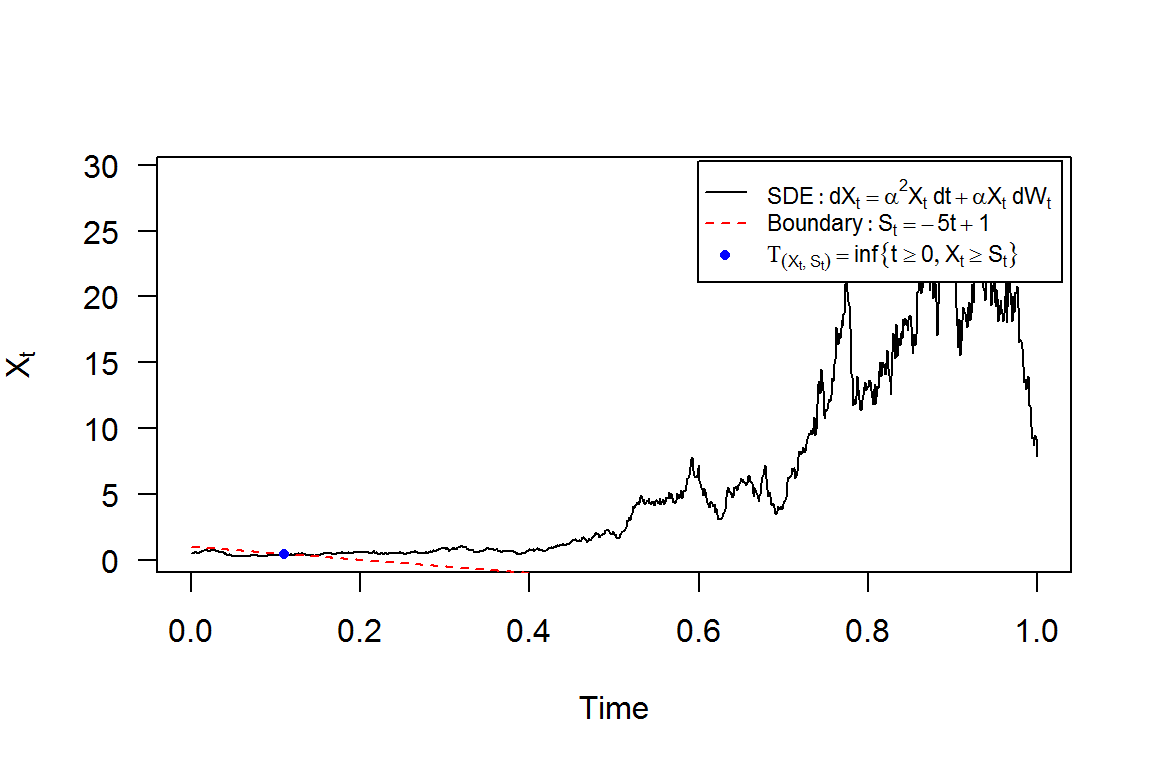The histograms of $$\tau_{S(t)}$$ with boundary $$S(t) = -5t+1$$, see e.g. Figure 2: the empirical distribution and kernel density of $$\tau_{S(t)}$$

require(MASS)
truehist(fpt1d$fpt,xlab = expression(tau[S(t)]) );box() lines(density(fpt1d$fpt),col="red",lwd=2)
legend("topright",c("Distribution histogram","Kernel Density"),inset =.01,pch=c(15,NA),lty=c(NA,1),col=c("cyan","red"),lwd=2,cex=0.8)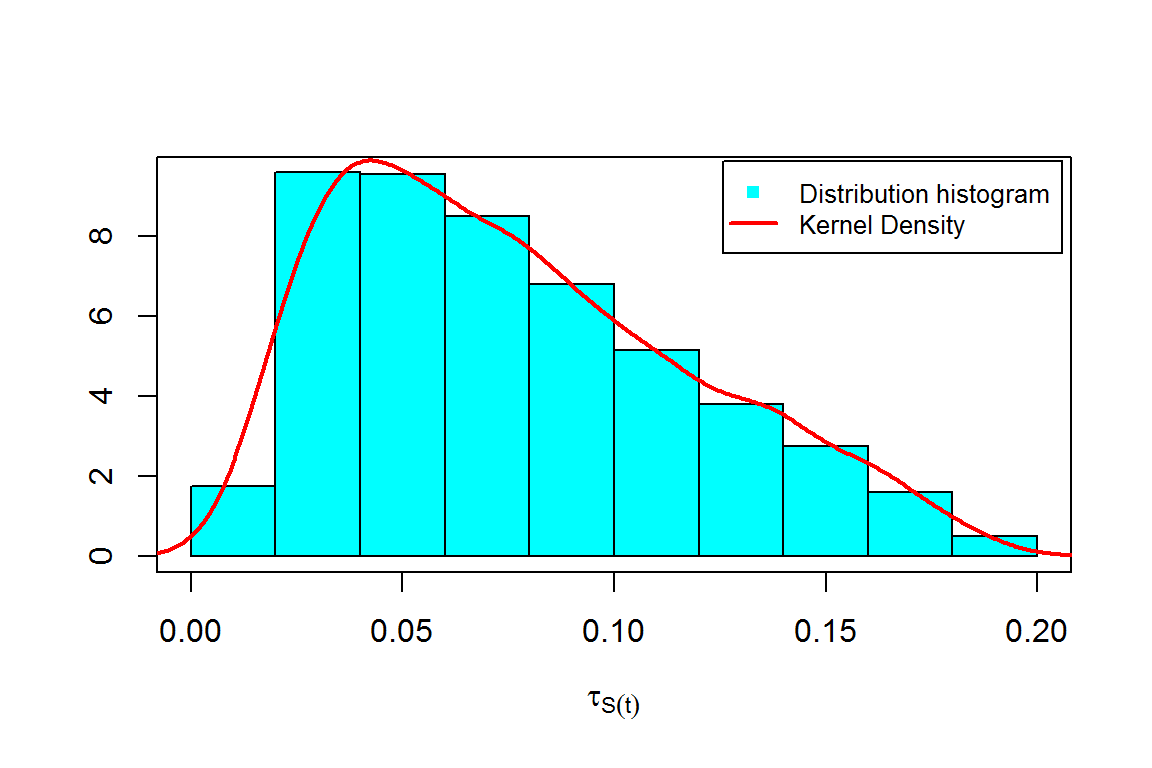## FPT for 2-Dim SDE’s

The following $$2$$-dimensional SDE’s with a vector of drift and a diagonal matrix of diffusion coefficients:

\begin{equation}\label{eq:09} \begin{cases} dX_t = f_{x}(t,X_{t},Y_{t}) dt + g_{x}(t,X_{t},Y_{t}) dW_{1,t}\\ dY_t = f_{y}(t,X_{t},Y_{t}) dt + g_{y}(t,X_{t},Y_{t}) dW_{2,t} \end{cases} \end{equation}

$$W_{1,t}$$ and $$W_{2,t}$$ is a two independent standard Wiener process. First passage time (2D) $$(\tau_{(S(t),X_{t})},\tau_{(S(t),Y_{t})})$$ is defined as:

$\left\{ \begin{array}{ll} \tau_{S(t),X_{t}}=\inf \left\{t: X_{t} \geq S(t)|X_{t_{0}}=x_{0} \right\} & \hbox{if} \quad x_{0} \leq S(t_{0}) \\ \tau_{S(t),Y_{t}}=\inf \left\{t: Y_{t} \geq S(t)|Y_{t_{0}}=y_{0} \right\} & \hbox{if} \quad y_{0} \leq S(t_{0}) \end{array} \right.$ and $\left\{ \begin{array}{ll} \tau_{S(t),X_{t}}= \inf \left\{t: X_{t} \leq S(t)|X_{t_{0}}=x_{0} \right\} & \hbox{if} \quad x_{0} \geq S(t_{0}) \\ \tau_{S(t),Y_{t}}= \inf \left\{t: Y_{t} \leq S(t)|Y_{t_{0}}=y_{0} \right\} & \hbox{if} \quad y_{0} \geq S(t_{0}) \end{array} \right.$

Assume that we want to describe the following SDE’s (2D):

\begin{equation}\label{eq016} \begin{cases} dX_t = 5 (-1-Y_{t}) X_{t} dt + 0.5 dW_{1,t}\\ dY_t = 5 (-1-X_{t}) Y_{t} dt + 0.5 dW_{2,t} \end{cases} \end{equation}

and $S(t)=-3+5t$

Set the system $$(X_t , Y_t)$$:

fx <- expression(5*(-1-y)*x) ; gx <- expression(0.5)
fy <- expression(5*(-1-x)*y) ; gy <- expression(0.5)
mod2d <- snssde2d(driftx=fx,diffx=gx,drifty=fy,diffy=gy,x0=2,y0=-2,M=1000)

Generate the couple $$(\tau_{(S(t),X_{t})},\tau_{(S(t),Y_{t})})$$, with fptsde2d() function::

St <- expression(-3+5*t)
fpt2d <- fptsde2d(mod2d, boundary = St)
names(fpt2d) ## names of output
##  "SDE"      "boundary" "fptx"     "fpty"
summary(fpt2d)
##
##   Monte-Carlo Statistics for the F.P.T of (X(t),Y(t))
## | T(S,X) = inf{t >=  0  : X(t) <=  -3 + 5 * t }
## | T(S,Y) = inf{t >=  0  : Y(t) <=  -3 + 5 * t }
##                      T(S,X)   T(S,Y)
## NA's                      0        0
## Mean                0.63154  0.59644
## Variance            0.00077  0.00079
## Median              0.63216  0.59602
## First quartile      0.61208  0.57843
## Third quartile       0.6503   0.6164
## Skewness           -0.01551 -0.08085
## Kurtosis             3.2649  2.81178
## Moment of order 3         0        0
## Moment of order 4         0        0
## Moment of order 5         0        0
## Int.conf Inf (95%)  0.57888  0.54179
## Int.conf Sup (95%)  0.68668  0.65066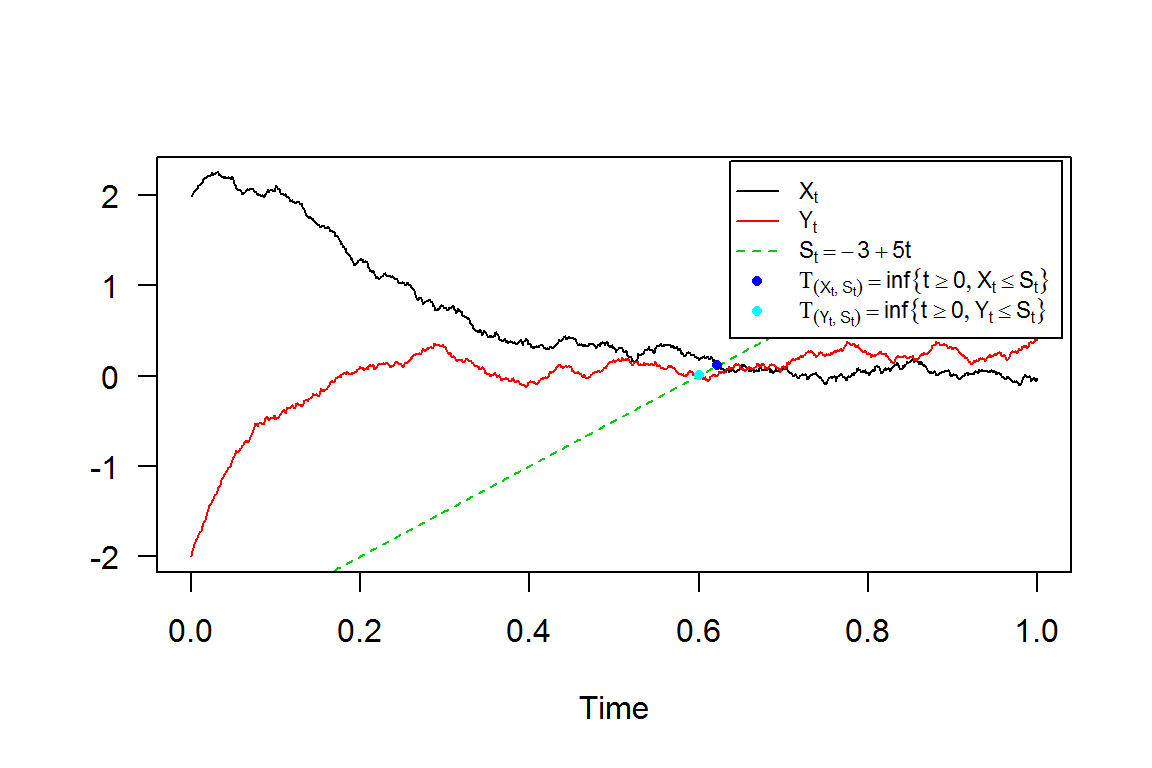Created using ggplot2 package plotted in (x, y)-space with dim = 2. A contour plot of density obtained from a realization of $$(\tau_{(S(t),X_{t})},\tau_{(S(t),Y_{t})})$$.

out <- data.frame(x=fpt2d$fptx,y=fpt2d$fpty)
library(ggplot2)
m <- ggplot(out, aes(x = x, y = y))
m + stat_density_2d(aes(fill = ..level..), geom = "polygon")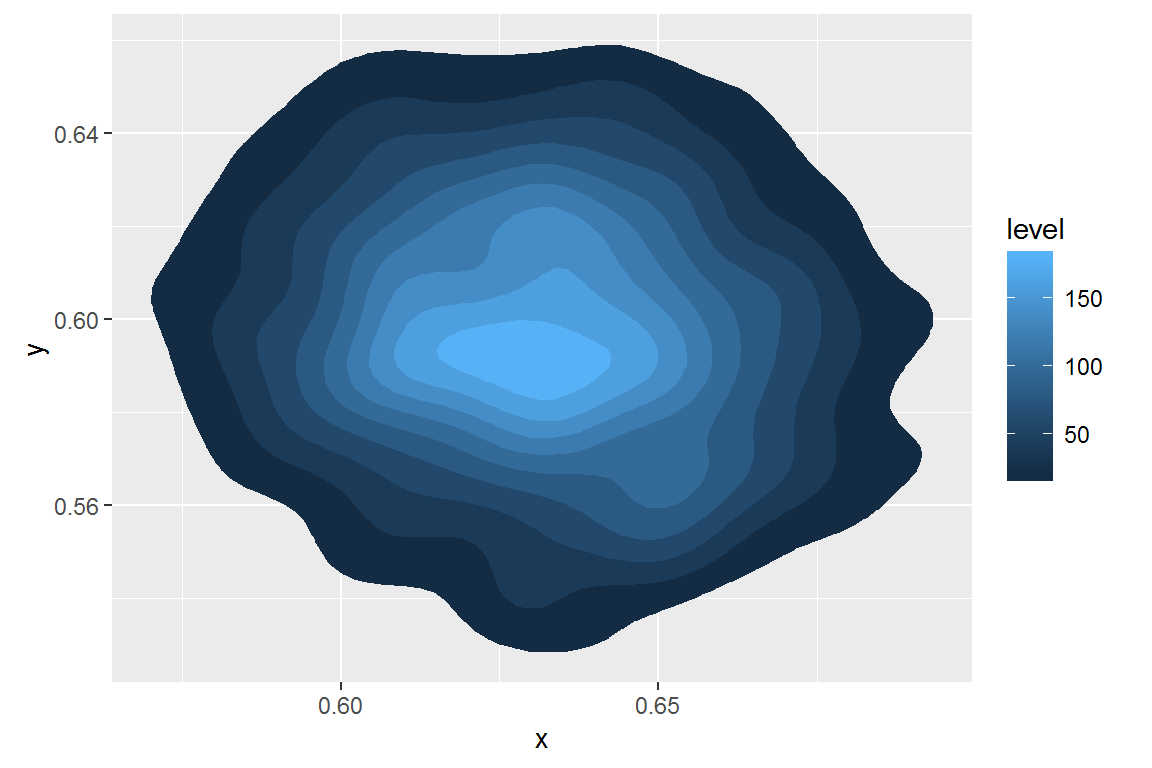A $$3$$D plot of the density obtained with sm, from a realization of $$(\tau_{(S(t),X_{t})},\tau_{(S(t),Y_{t})})$$.

library(sm)
sm.density(out,display="persp")
## Warning: weights overwritten by binning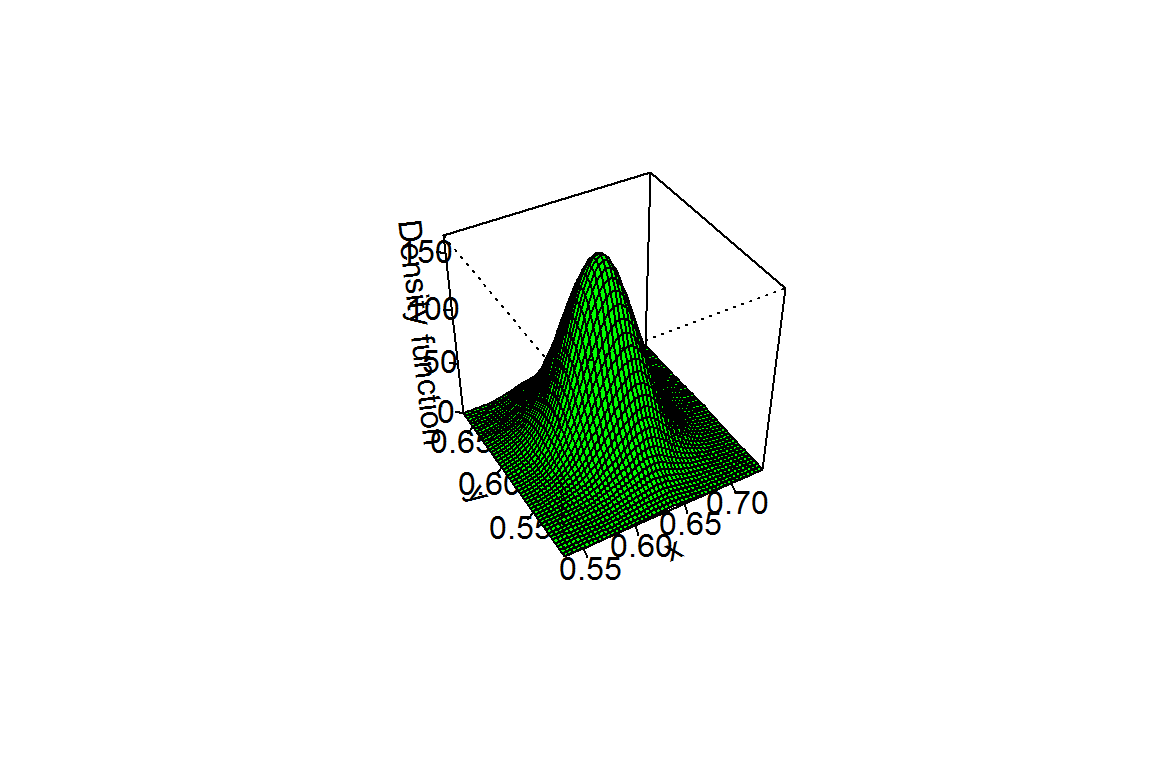## FPT for 3-Dim SDE’s

The following $$3$$-dimensional SDE’s with a vector of drift and a diagonal matrix of diffusion coefficients:

Ito form: \begin{equation}\label{eq17} \begin{cases} dX_t = f_{x}(t,X_{t},Y_{t},Z_{t}) dt + g_{x}(t,X_{t},Y_{t},Z_{t}) dW_{1,t}\\ dY_t = f_{y}(t,X_{t},Y_{t},Z_{t}) dt + g_{y}(t,X_{t},Y_{t},Z_{t}) dW_{2,t}\\ dZ_t = f_{z}(t,X_{t},Y_{t},Z_{t}) dt + g_{z}(t,X_{t},Y_{t},Z_{t}) dW_{3,t} \end{cases} \end{equation}

$$W_{1,t}$$, $$W_{2,t}$$ and $$W_{3,t}$$ is a 3 independent standard Wiener process. First passage time (3D) $$(\tau_{(S(t),X_{t})},\tau_{(S(t),Y_{t})},\tau_{(S(t),Z_{t})})$$ is defined as:

$\left\{ \begin{array}{ll} \tau_{S(t),X_{t}}=\inf \left\{t: X_{t} \geq S(t)|X_{t_{0}}=x_{0} \right\} & \hbox{if} \quad x_{0} \leq S(t_{0}) \\ \tau_{S(t),Y_{t}}=\inf \left\{t: Y_{t} \geq S(t)|Y_{t_{0}}=y_{0} \right\} & \hbox{if} \quad y_{0} \leq S(t_{0}) \\ \tau_{S(t),Z_{t}}=\inf \left\{t: Z_{t} \geq S(t)|Z_{t_{0}}=z_{0} \right\} & \hbox{if} \quad z_{0} \leq S(t_{0}) \end{array} \right.$ and $\left\{ \begin{array}{ll} \tau_{S(t),X_{t}}= \inf \left\{t: X_{t} \leq S(t)|X_{t_{0}}=x_{0} \right\} & \hbox{if} \quad x_{0} \geq S(t_{0}) \\ \tau_{S(t),Y_{t}}= \inf \left\{t: Y_{t} \leq S(t)|Y_{t_{0}}=y_{0} \right\} & \hbox{if} \quad y_{0} \geq S(t_{0}) \\ \tau_{S(t),Z_{t}}= \inf \left\{t: Z_{t} \leq S(t)|Z_{t_{0}}=z_{0} \right\} & \hbox{if} \quad z_{0} \geq S(t_{0}) \\ \end{array} \right.$

Assume that we want to describe the following SDE’s (3D): \begin{equation}\label{eq0166} \begin{cases} dX_t = 4 (-1-X_{t}) Y_{t} dt + 0.2 dW_{1,t}\\ dY_t = 4 (1-Y_{t}) X_{t} dt + 0.2 dW_{2,t}\\ dZ_t = 4 (1-Z_{t}) Y_{t} dt + 0.2 dW_{3,t} \end{cases} \end{equation}

and $S(t)=-3+5t$

Set the system $$(X_t , Y_t , Z_t)$$:

fx <- expression(4*(-1-x)*y) ; gx <- expression(0.2)
fy <- expression(4*(1-y)*x)  ; gy <- expression(0.2)
fz <- expression(4*(1-z)*y)  ; gz <- expression(0.2)
mod3d <- snssde3d(driftx=fx,diffx=gx,drifty=fy,diffy=gy,driftz=fz,diffz=gz,x0=2,y0=-2,z0=0,M=1000)

Generate the triplet $$(\tau_{(S(t),X_{t})},\tau_{(S(t),Y_{t})},\tau_{(S(t),Z_{t})})$$, with fptsde3d() function::

St <- expression(-3+5*t)
fpt3d <- fptsde3d(mod3d, boundary = St)
names(fpt3d) ## names of output
##  "SDE"      "boundary" "fptx"     "fpty"     "fptz"
summary(fpt3d)
##
##  Monte-Carlo Statistics for the F.P.T of (X(t),Y(t),Z(t))
## | T(S,X) = inf{t >=  0  : X(t) <=  -3 + 5 * t }
## | T(S,Y) = inf{t >=  0  : Y(t) >=  -3 + 5 * t }
## | T(S,Z) = inf{t >=  0  : Z(t) <=  -3 + 5 * t }
##                     T(S,X)  T(S,Y)   T(S,Z)
## NA's                     0       0        0
## Mean               0.58147 0.78676   0.7695
## Variance           0.00013 0.00122  0.00029
## Median             0.58138 0.78253  0.76943
## First quartile     0.57342 0.76354   0.7578
## Third quartile     0.58941 0.80572  0.78135
## Skewness           0.11006 1.18629 -0.08683
## Kurtosis           2.86346 6.33489  3.12204
## Moment of order 3        0   5e-05        0
## Moment of order 4        0   1e-05        0
## Moment of order 5        0       0        0
## Int.conf Inf (95%) 0.56067 0.73227  0.73681
## Int.conf Sup (95%) 0.60318  0.8662  0.80184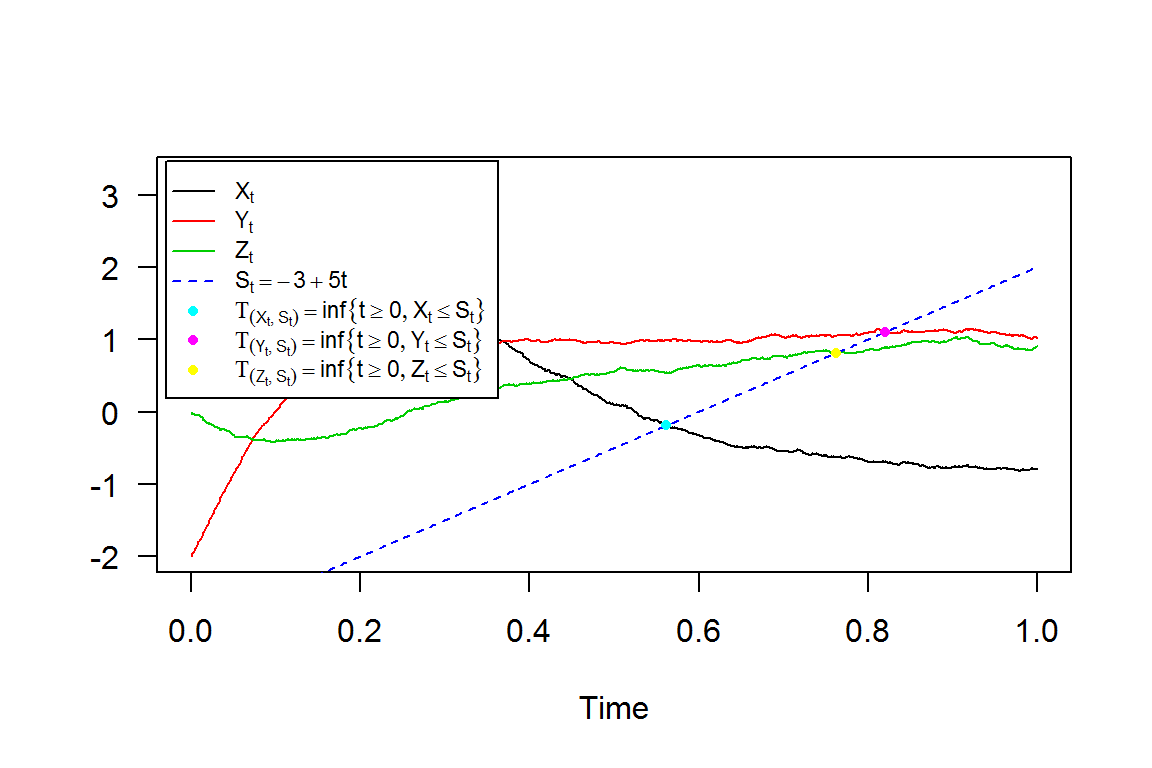A $$3$$D plot of the density obtained with sm, from a realization of $$(\tau_{(S(t),X_{t})},\tau_{(S(t),Y_{t})},\tau_{(S(t),Z_{t})})$$.

out <- data.frame(x=fpt3d$fptx,y=fpt3d$fpty,z=fpt3d\$fptz)
library(sm)
sm.density(out,display="rgl")